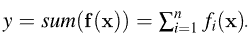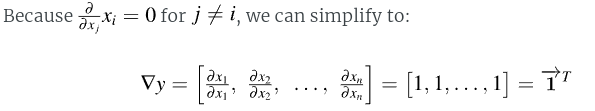# The Matrix Calculus You Need For Deep Learning - Sec 4.2 and 4.4

I started reading the paper that Jeremy and Terence wrote on Matrix Calculus for DL last night but am having a problem understanding the following:

1. In section 4.2 where it covers derivatives of scalar-vector products there’s a line that says:

"We know that element-wise operations imply that f_t is purely a function of w_i and g_i is purely a function of x_i." I am very confused about this claim. It might be explained in the text already but I don’t think I understand why that has to be true? Since, f is function of multiple parameters (that we all group into the w vector) why can we not have f_1 = w_1 * w_2?

1. In section 4.4 it talks about summing over the vector of functions i.e. sum(f(x)) but then later on it says “Let’s look at the gradient of the simple y = sum(x). The function inside the summation is just f_i(x) = x_i” and also “f_i(zx) = zx_i.”

I don’t quite understand why is f_i again only dependent on one of the components of x?

Thank you.

I think you are thinking about a general multi-parameters function but in this case, we are considering only the element-wise operation.

Hey, thanks for the reply. Could you elaborate a little bit on how that implies what the paper is saying? Like can you expand on why “we are considering only the element-wise operation.” leads to "f_i(x) = x_i” and also “f_i(zx) = zx_i.”

I think the general vector sum reduction is as below:But we expand only for the case of y = sum(x) (  Let's look at the gradient of the simple y = sum(x) )

So: y = x1 + x2 + x3 + … + xi + …

As you can see f_i(x) is only x_i

So the gradient of y is:In which part of these expressions you’re still not clear? Hope it helps

1 Like

Ah! I see okay that’s what they are doing. Okay I get it now. Thanks a lot

Do you by any chance know anything about the first question

This is the definition of element-wise operation :). the i_th output depends only on the i_th inputs

1 Like

Not related to your questions, but a useful tip for writing out math equations so they’re easier to read: if you surround your equations with a single $symbol, the equation will be rendered to look proper. For example, $y = mx + c$ will be rendered as y = mx + c. • $f_{1} = w_{1} \cdot w_{2}$f_{1} = w_{1} \cdot w_{2} • $f_{1} = w_{1} \times w_{2}$f_{1} = w_{1} \times w_{2} • $y = \text{sum}(x)\$y = \text{sum}(x)

Here’s a cheat sheet for how to write most LaTeX symbols: LaTeX Math Symbols Cheat Sheet - Kapeli

1 Like

I didn’t know this supported LaTeX thanks1 Like